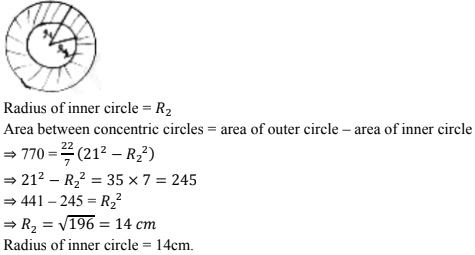# Chapter 15 Areas Related to Circles RD Sharma Solutions Exercise 15.1 Class 10 Maths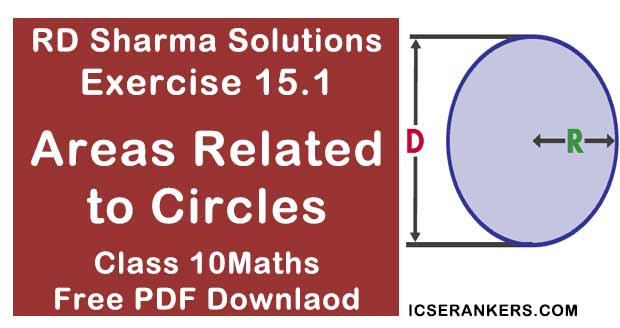Chapter Name RD Sharma Chapter 15 Areas Related to Circles Book Name RD Sharma Mathematics for Class 10 Other Exercises Exercise 15.2Exercise 15.3Exercise 15.4 Related Study NCERT Solutions for Class 10 Maths

### Exercise 15.1 Solutions

1. Find the circumference and area of circle of radius 4.2 cm .

Solution

Circumference = 2 × r2. Find the circumference of a circle whose area is 301.84 cm2 .

Solution
Area of circle  = 301.84cm2 .
Area of circle = πr2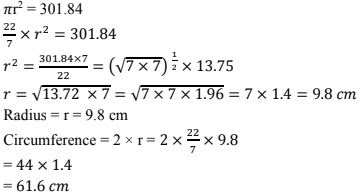3. Find the area of circle whose circumference is 44 cm.
Solution
Circumference = 44 cm
Circumference = 2 × r = 44 cm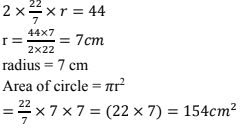4. The circumference of a circle exceeds diameter by 16.8 cm. Find the circumference of circle.
Solution
Let radius of circle = r cm
Diameter (d) = 2× radius  = 2r
Circumference (c) = 2πr
Given circumference exceeds diameter by 16.8 cm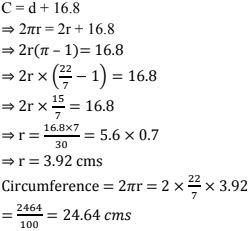5. A horse is tied to a pole with 28m long string. Find the area where the horse can graze.
Solution
Length of string l = 28m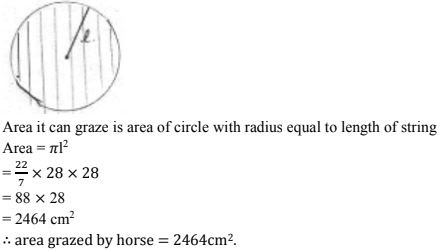6. A steel wire when bent is the form of square encloses an area of 12 cm2 . If the same wire is bent in form of circle. Find the area of circle.
Solution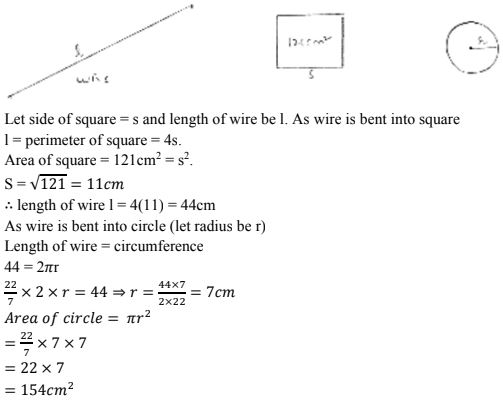7. A horse is placed for grazing inside a rectangular field 40 m by 36 m and is tethered to one corner by a rope 14m long. Over how much area can it graze.
SolutionThe fig shows rectangular field ABCD at corner A, a horse is tied with rope length = 14 m.
The area it can graze is represented A as shaded region = area of quadrant with (radius = length) of string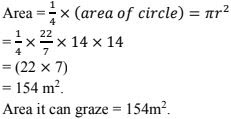8. A sheet of paper is in the form of rectangle ABCD in which AB = 40 cm and AD = 28 cm.
A semicircular portion with BC as diameter is cut off. Find the area of remaining paper.
Solution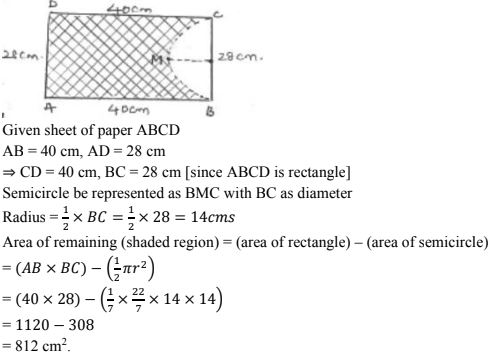9. The circumference of two circles are in ratio 2 : 3. Find the ratio of their areas
Solution
Let radius of two circles be r1 and r2 then their circumferences will be 2πr1 : 2πr2 = r1 : r2
But circumference ratio is given as 2 : 3
r1 : r2 = 2 : 3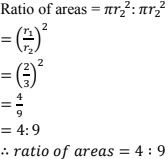10. The side of a square is 10 cm. find the area of circumscribed and inscribed circles.
Solution
Circumscribed circle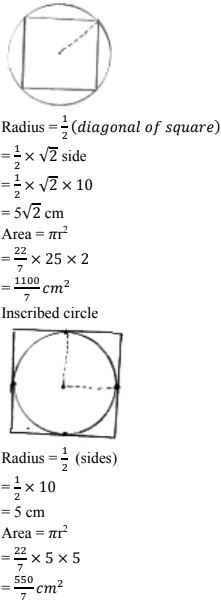11. The sum of the radii of two circles is 140 cm and the difference of their circumferences in 88 cm. Find the diameters of the circles.
Solution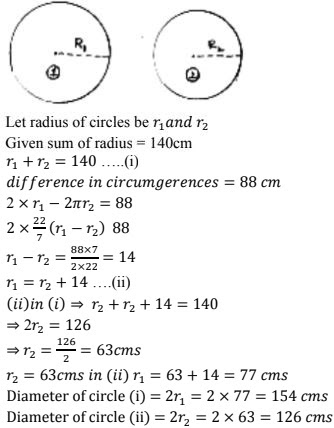12. The area of circle, inscribed in equilateral triangle is 154 cm2 . Find the perimeter of triangle.
Solution13. A field is in the form of circle. A fence is to be erected around the field. The cost of fencing would to Rs. 2640 at rate of Rs. 12 per metre. Then the field is to be thoroughs ploughed at cost of Rs. 0.50 per m2 . What is amount required to plough the field ?
Solution
Given,
Total cost of fencing the circular field = Rs. 2640
Cost per metre fencing = Rs. 12
Total cost of fencing = circumference × cost per fencing
⇒2640 = circumference × 12
⇒ circumference = 2640/12 = 220 m
Let radius of field be r m
Circumference = 2πr m
2πr = 220
2 × 22/7 × r = 220
r = 70/2 = 35m
Area of field = πr2 .
= 22/7 × 35×35
= 3850 m2 .
Cost of ploughing per m2 land = Rs. 0.50
Cost of ploughing 3850 m2 land = 1/2 × 3850 = Rs. 1925.

14. If a square is inscribed in a circle, find the ratio of areas of the circle and the square.
Solution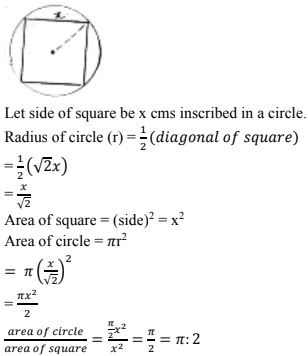15. A park is in the form of rectangle 120m × 100m. At the centre of park there is a circular lawn. The area of park excluding lawn is 8700m2 . Find the radius of circular lawn.
Solution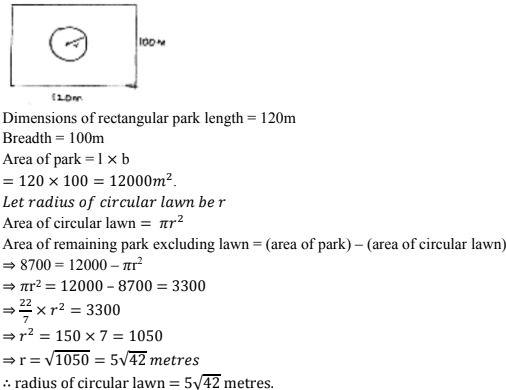16. The radii of two circles are  8cm and 6cm respectively. Find the radius of the circle having its are equal to the sum of the areas of two circles.
Solution
Radius of circles are 8 cm and 6 cm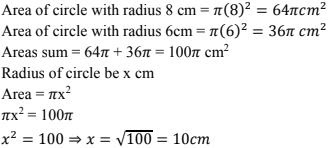17. The radii of two circles are 19cm and 9 cm respectively. Find the radius and area of the circle which has circumferences is equal to sum of circumference of  two circles.
Solution
Radius of 1st circle = 19cm
Radius of 2nd circle = 9cm
Circumference of 1st circle = 2(19) = 38π cm
Circumference of 2nd circle = 2π(9) = 18π cm
Let radius of required circle = R cm18. A car travels 1 km distance in which each wheel makes 450 complete revolutions. Find the radius of wheel.
Solution
Let radius of wheel = 'r' m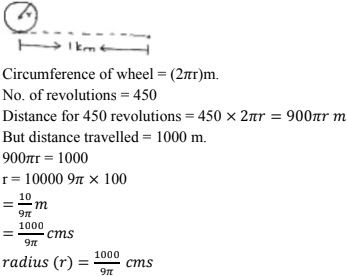19. The area enclosed between the concentric circles is 770 cm2 . If the radius of inner circle.
Solution
Radius of outer circle = 21 cm